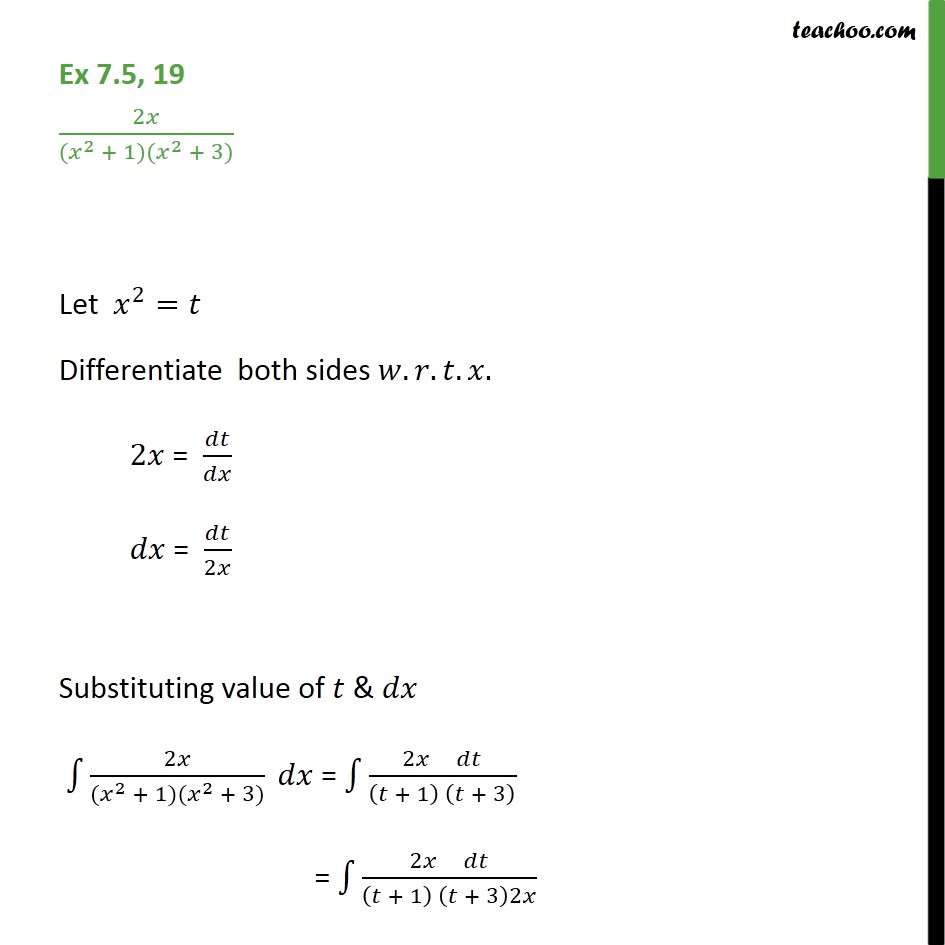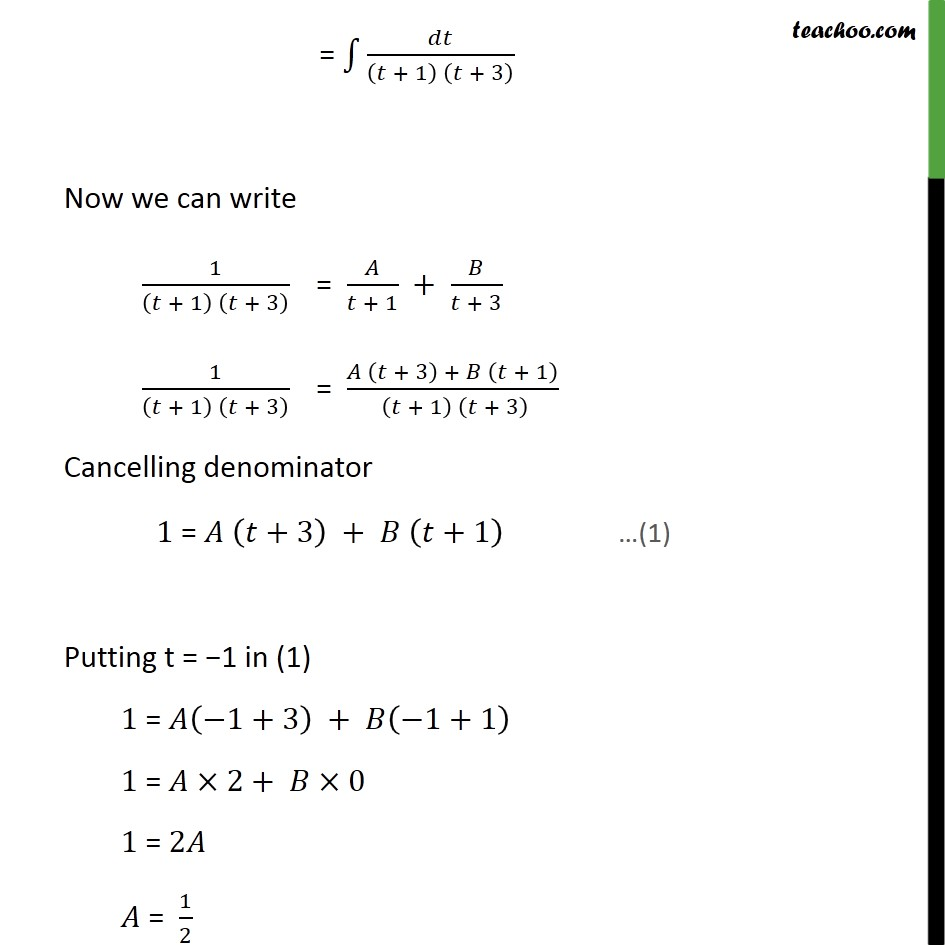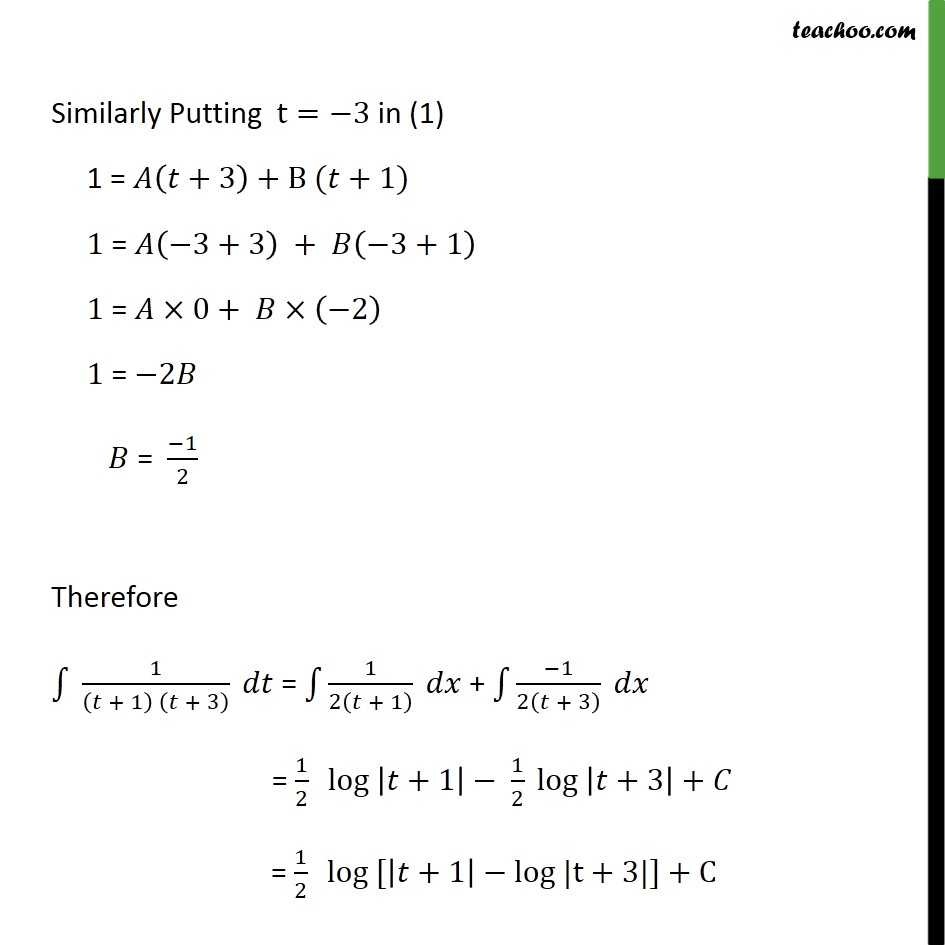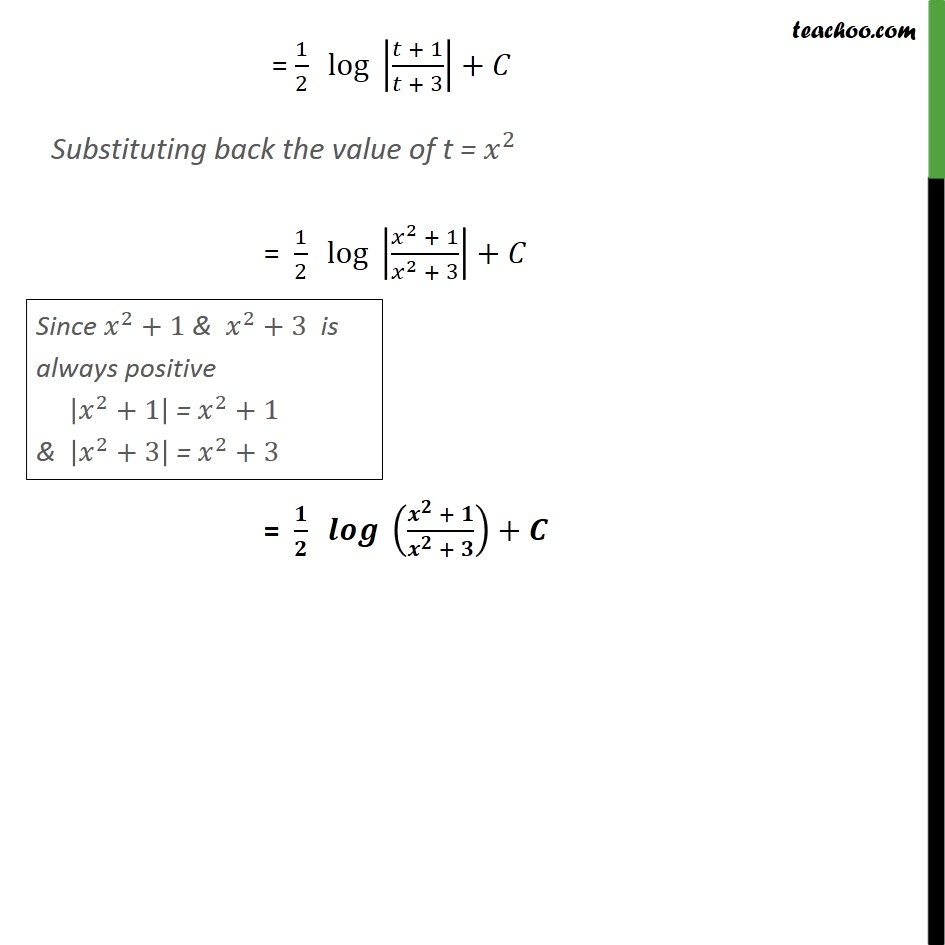Integration by partial fraction - Type 1

Chapter 7 Class 12 Integrals
Concept wiseIntroducing your new favourite teacher - Teachoo Black, at only ₹83 per month

### Transcript

Ex 7.5, 19 2 ( 2 + 1)( 2 + 3) Let 2 = Differentiate both sides . . . . 2 = = 2 Substituting value of & 2 ( 2 + 1)( 2 + 3) = 2 + 1 + 3 = 2 + 1 + 3 2 = + 1 + 3 Now we can write 1 + 1 + 3 = + 1 + + 3 1 + 1 + 3 = + 3 + + 1 + 1 + 3 Cancelling denominator 1 = +3 + +1 Putting t = 1 in (1) 1 = 1+3 + 1+1 1 = 2+ 0 1 = 2 = 1 2 Similarly Putting t= 3 in (1) 1 = +3 + B ( +1) 1 = 3+3 + 3+1 1 = 0+ 2 1 = 2 = 1 2 Therefore 1 + 1 + 3 = 1 2 + 1 + 1 2 + 3 = 1 2 log +1 1 2 log +3 + = 1 2 log [ +1 log |t+3|]+C = 1 2 log + 1 + 3 + Substituting back the value of t = 2 = 1 2 log 2 + 1 2 + 3 + = + + +